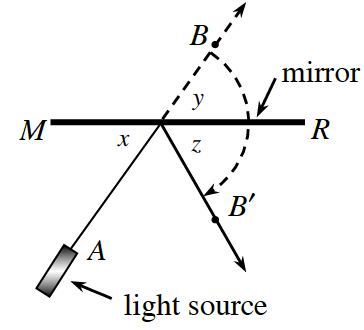### Home > CCG > Chapter 3 > Lesson 3.2.5 > Problem3-102

3-102.

A laser light is pointed at a mirror as shown at right. If $∠x$ measures $48º$, what are the measures of $∠y$ and $∠z$? Justify your reasons.

The angle of incidence is equal to the angle of reflection.

$∠x$ and $∠y$ are vertical angles.